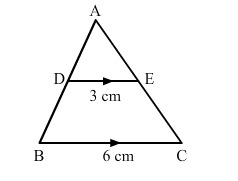# In the given figure, DE ∥ BC. If DE = 3 cm, BC = 6 cm and ar(∆ADE) = 15 cm2,Question:

In the given figure, DE ∥ BC. If DE = 3 cm, BC = 6 cm and ar(∆ADE) = 15 cm2, find the area of ∆ABC.Solution:

It is given that DE ∥ BC

$\therefore \angle A D E=\angle A B C$ (Corresponding angles)

$\angle A E D=\angle A C B$ (Corresponding angles)

By AA similarity, we can conclude that $\triangle A D E \sim \triangle A B C$.

$\therefore \frac{\operatorname{ar}(\Delta A D E)}{\operatorname{ar}(\Delta A B C)}=\frac{D E^{2}}{B C^{2}}$

$\Rightarrow \frac{15}{\operatorname{ar}(\Delta A B C)}=\frac{3^{2}}{6^{2}}$

$\Rightarrow \operatorname{ar}(\Delta A B C)=\frac{15 \times 36}{9}$

$=60 \mathrm{~cm}^{2}$

Hence, area of triangle ABC is 60 cm2.​